# Matplotlib in Python: Explained Various Plots with Examples

## What is matplotlib?

Out of the several libraries available in Python, matplotlib in python is one such visualization library that helps in the visualization of 2D plots of an array. The data visualization library is built on NumPy arrays. It was in the year 2002, that the multi-platform data visualization library was introduced by John Hunter. The library offers visualization of data and the graphical plotting of the data providing an alternative to MATLAB. Matplotlib’s APIs i.e. Application Programming Interfaces which are used by the developers to embed plots in GUI applications.

Several graphical plots like bar, line, histogram, scatter, etc. are offered by matplotlib. The visualization offered by the matplotlib plot allows access to huge amounts of data visually. The visual data plot can be generated through a code of few lines only due to the structured nature of a python matplotlib script.

Two APIs are used for overlaying the matplotlib scripting layer:

• Python API: It is a hierarchy of python code objects.
• OO (Object Oriented) API: A direct access to the backend layers of Matplotlib is provided by the API.

### Installation

The installation of the matplotlib library can be done through downloading of matplotlib and its dependencies from the Python Package Index (PyPI) as a binary package.

The command that can be used for installing the library is

python -m pip install matplotlib

In an operating system like Windows, Linux, and macOS, matplotlib and its dependencies are present as wheel packages. In such cases the command to be executed is.

python -mpip install -U matplotlib

The library is also available as uncompiled source files whose installation is fairly complex as the local system will require having the appropriate compiler for the OS. Also, the ActiveState Platform may be used for building matplotlib from source and package it for the required OS.

### Importing

The importing of the matplotlib in python is carried out through the commands

• from matplotlib import pyplot as plt
• import matplotlib.pyplot as plt

## Various Plots and Examples

The Matplotlib UI Menu is generated when plots are created through the Matplotlib. Customization of the plot and toggling of elements along with the ability to zoom into the plots are offered by the Matplotlib UI.

### 2. Matplotlib and NumPy

The NumPy is a package under python for carrying out scientific computations. Matplotlib is built over the NumPy and it uses the functions provided by NumPy for its numerical data and multi-dimensional arrays.

### 3. Matplotlib and Pandas

Pandas is a library of python that is used for the manipulation of data and analysis by matplotlib. It is not a required dependency for the matplotlib but provides a data frame.

Matplotlib plots allow the visual representation of  huge volumes of data. With the plots, the trends and specific patterns present in data could be identified which is essential for making correlations. Matplotlib Plots basically provide a way for reasoning about quantitative information.

### Some of the types of matplotlib plots are:

#### 1. Line plot:

Using two points

• The Matplotlib Line Plot is generated through importing pyplot.
• For drawing points in a diagram the plot() function is used which by default draws a line from one point to another.
• Two parameters are taken into account that specify the points for drawing the line.
• X-axis points are stored as array in Parameter 1.
• Y-axis points are stored as array in Parameter 2.
• Example: If a line has to be plotted from points (2, 6), to (10, 15), then two arrays have to be passed i.e. [2, 10], and [6, 15].

Example: A code showing the plotting of lines and the generated plot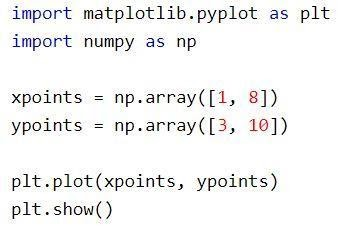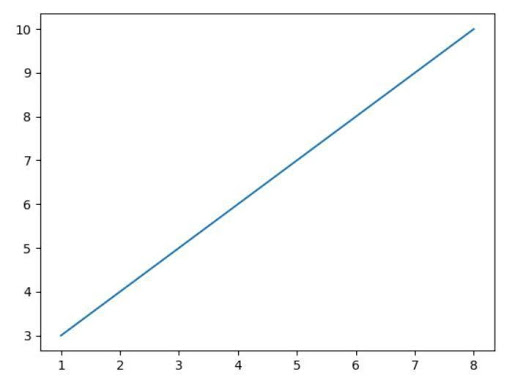Source

#### 2. Using Multiple Points

• Like the way two points are used for plotting, multiple points are allowed to be plotted using matplotlib in python.
• The points should be in the same number in both the axes for plotting a number of points.
• Input: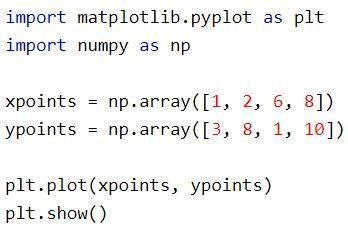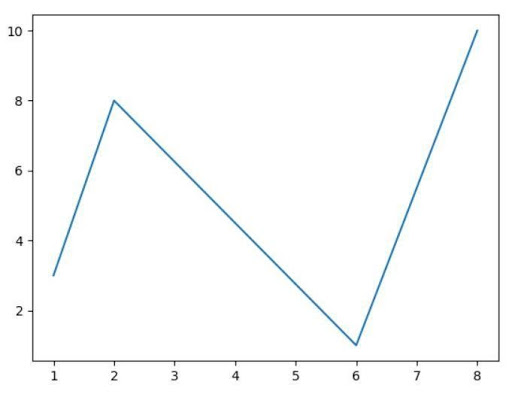Source

#### 3. Line points without x axis points

• If X-axis points are not specified, then default values for X-axis are taken based on the Y-axis points.
• Input: The code will remain the same like the above codes for plotting lines but with only one array as input, i.e. an array for the Y-axis. The X-axis will be taken as default.

ypoints = np.array([10, 8, 12, 20, 3, 9])

• Plot generated: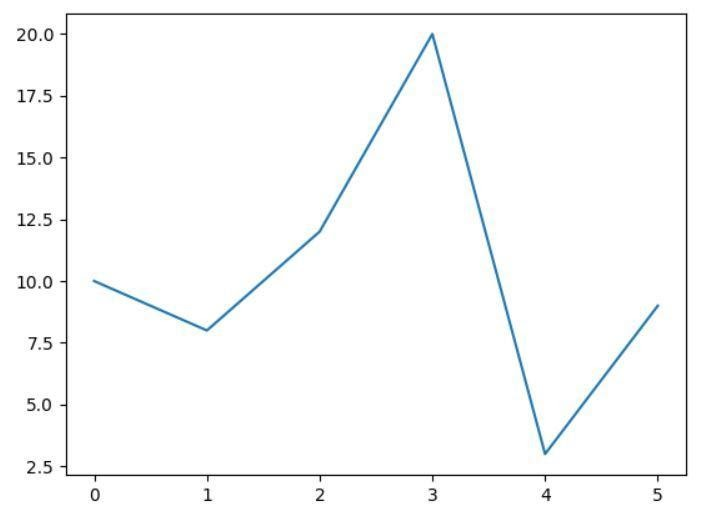### Various options are present in the matplotlib that allows increasing the visual effects of the plots:

#### 1. Markers

• To enhance the visual effects of the points in a diagram, a specified marker can be used using the keyword marker.
• The markers can be a star, Circle, Point, Pixel, X, etc.
• Example: plt.plot(ypoints, marker = ‘o’) can be used for plotting points
• The other lists of markers are shown in the below snippet taken from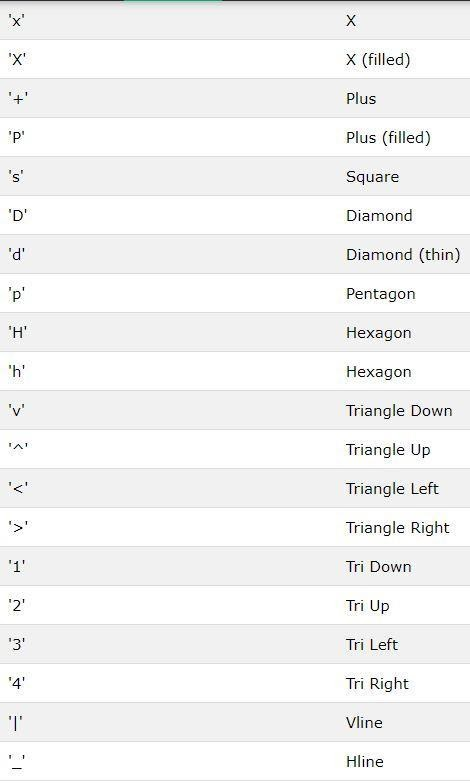Source

• The marker can be changed according to color (140 supported colors), size, and the type of line that can be used like dotted, solid, or dashed line.
• markeredge (mec) and markerfacecolor (mfc) commands are used to color the entire marker.
• It offers the option of coloring only the edge of the marker or the whole marker.
• Markersize or in short ms is used for setting the size of the marker.

Syntax:  plt.plot(ypoints, marker = ‘o’, ms = 30)

#### 2. Matplotlib Line

• The style of the plotted line can be changed accordingly with the options of linestyle, dotted, or dashed represented as ls, :, or .

Syntax: plt.plot(ypoints, ls = ‘:’)

• The color of the line can be changed accordingly with the keyword color or in a shorter form using c. matplotlib provides 140 supported colors for changing the color appearance of the line.
• The width of the line can be changed with the argument linewidth or lw. It is a floating number in points.
• Multiple lines can be plotted in the same graph using plt.plot() functions.
• grid() function is used for adding grid lines into the plot. Axis parameters can be added to specify in which axis the grid line is required.

Syntax: plt.grid(axis = ‘x’)

• Properties of the grid can be changed accordingly like color, style of line and width through the arguments, color, linestyles, and number.

Syntax: plt.grid(color = ‘green’, linestyle = ‘–‘, linewidth = 0.5)

#### 3. Matplotlib Labels and titles

• xlabel() and ylabel() functions are used for labeling the respective asex.
• title() function is used for setting up a title for the plot.
• Font properties of the plot can be changed with the fontdict parameter.
• The loc parameter can be used to specify the position of the title.

Multiple plots can be drawn in one figure using the subplots() function.

#### 4. Matplotlib Scatter Plot

• The scatter() function can be used with pyplot to draw a scatter plot.
• Two arrays of the same length are required, i.e. one array for each axis.
• Example: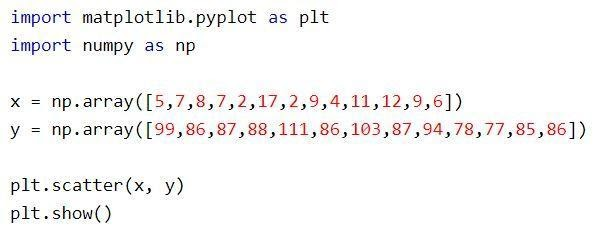Source

Plot generated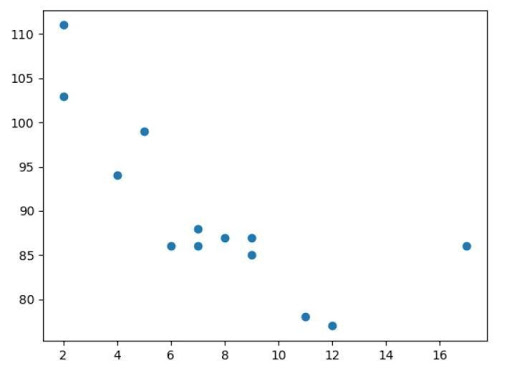• color or the c argument is used to color the points in the scatter plot.
• Colormap can be used to specify the required color in the scatterplot. Each color in the colormap has a specific value. It can be included through the argument cmap nd then assigning the name of the colormap. Several in=built colormaps are available in matplotlib.

Syntax: plt.scatter(x, y, c=colors, cmap=’viridis’)

Viridis is an in-built colormap available in matplotlib.

• Size and transparency of the dots can be changed through the s and the alpha argument.
• The colormap can be combined with different sizes of the dots.

#### 5. Matplotlib Bar diagrams

• bar() function are used for drawing the bar diagrams. The arguments for the layout of the bars are mentioned in the bar() function. It plots vertical bar diagrams.

• For plotting horizontal bar diagrams the barh() function is used.
• Input: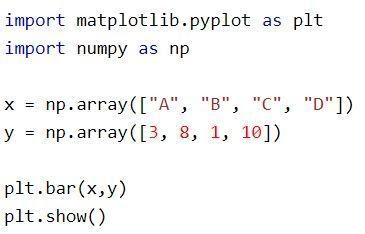• Plot generated: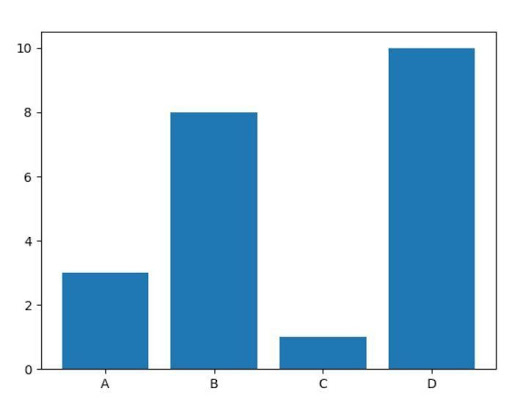Source

• The color argument is used with the bar() and barh() function to set the bar colors.

Syntax: plt.bar(x, y, color = “green”).

• The width argument is used with the bar() and barh() function to set the bar width.

Syntax: plt.bar(x, y, width = 0.2).

• Another argument taken up by the bar() and barh() function is height which is used to set the bar height.

#### 6. Matplotlib Pie plot

• A pie chart is created through the pie() function in the matplotlib library.
• Example: Input: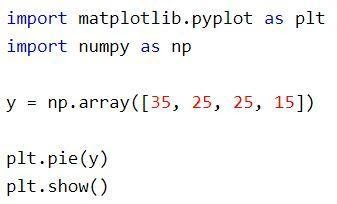• Plot generated: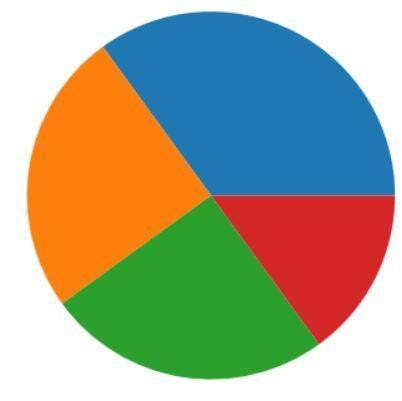• Each wedge can be labeled with the parameter label which is an array with the labels for each wedge.

Syntax: mylabels = [“cars”“bikes”“cycles”“buses”]

• Default start angle in a pie chart is the X-axis, which can be changed with the parameter startangle. The angle is defined in degrees and the default angle is 0.
• With the explode parameter, the required wedge can be displayed to be standing out. It is specified through an array with the value of the wedge to be standing out and the rest values kept as 0.

Syntax: myexplode = [0.2, 0, 0, 0]

• Setting shadows parameter to true will create a shadow for the pie chart.
• colors parameter is used to specify the colors of each wedge through an array.

Syntax:  mylabels = [“cars”“bikes”“cycles”“buses”]

mycolors = [“black”, “hotpink”, “blue”, green””]

• legend() function is used to add an explanation to each wedge.

#### 7. Histogram

• Histogram is used for plotting the frequency distributions.
• hist() function is used for creating a histogram that uses an array of numbers for creating the histogram.
• Example: Input: the above lines will be the same as that used for plotting bar diagrams.

x = np.random.normal(90, 100, 200)

print(x)

• Plot generated: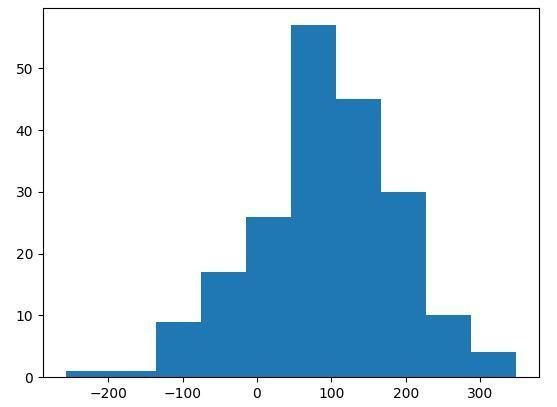## Conclusion

As discussed in the article, matplotlib in python can be used for the plotting of the data in various styles. Further various options are available to enhance our plots allowing the user to label, resize, and color as per their wish. Therefore, python and its libraries are quite helpful for the analysis and handling of data in the present age.

Python programming training over the field of data science is available in the course Executive PG Programme in Data Science offered by upGrad. If you are willing to get trained under industry experts and explore the various opportunities held by data science, you can enroll in the course.  The course is offered by IIIT-Bangalore and designed especially for Entry to mid-level professionals within the age group of 21 to 45 years. Irrespective of any gender, if you fall within this mentioned category and dream of becoming a leading data scientist, come join us in this venture. For any assistance ship, our team is ready to help you.## 0 replies on “Matplotlib in Python: Explained Various Plots with Examples”